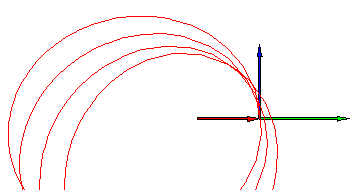Visualizing the Laplace-Runge-Lenz Vector - Maple Application Center
Application Center Applications Visualizing the Laplace-Runge-Lenz Vector

## Visualizing the Laplace-Runge-Lenz Vector

Author
: Frank WangThis Application runs in Maple. Don't have Maple? No problem!
The vector treatment of Kepler's first law presented in most calculus textbooks is based on the existence of a constant vector that is associated with the exact inverse square force law. Such a treatment is not a general substitute for the methods based on the differential equations of motion. This worksheet demonstrates how to use Maple to solve the differential equations governing planetary motion, and how to visualize the Laplace-Runge-Lenz vector which is peculiar to the force law of the form k/r^2.

#### Application Details

Publish Date: March 02, 2009
Created In: Maple 12
Language: English

#### Share

This app is not in any Collections

vector-calculus

1
1
1